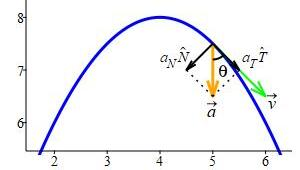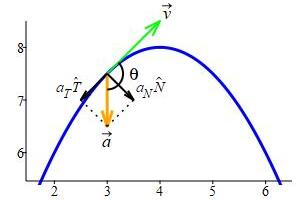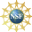# 8. Properties of Curves

## g. Tangential and Normal Acceleration

The acceleration of a curve, $$\vec{a}$$, measures the rate of change of the velocity, $$\vec{v}$$, which has a magnitude and a direction. The magnitude of the velocity, $$|\vec{v}|$$, is the speed the curve, $$speed=\dfrac{ds}{dt}=|\vec{v}|$$, while the direction of the velocity, $$\hat{v}$$, is the unit tangent vector, $$\hat{T}=\hat{v}$$. There is a way to split up the acceleration so that one part measures the rate of change of the speed while the other part measures the rate of change of the unit tangent vector.

Since $$\vec{a}$$ lies in the plane of $$\hat{T}$$ and $$\hat{N}$$, we know $$\vec{a}$$ can be written as a linear combination of $$\hat{T}$$ and $$\hat{N}$$: $\vec{a}=a_T\,\hat{T}+a_N\,\hat{N}$ The coefficient $$a_T$$ is called the tangential acceleration and the coefficient $$a_N$$ is called the normal acceleration. These are the scalar projections (components) of $$\vec{a}$$ parallel and perpendicular to $$\vec{v}$$: \begin{aligned} a_T\hat{T}=\text{proj}_{\vec{v}}\vec{a} =\dfrac{\vec{a}\cdot\vec{v}}{|\vec{v}|^2}\vec{v} &\qquad \text{and} \qquad a_N\hat{N}=\text{proj}_{\bot\vec{v}}\vec{a} =\vec{a}-\,\dfrac{\vec{a}\cdot\vec{v}}{|\vec v|^2}\vec{v} \\ a_T=\text{comp}_{\vec{v}}\vec{a} =\dfrac{\vec{a}\cdot\vec{v}}{|\vec{v}|} &\qquad \text{and} \qquad a_N=\text{comp}_{\bot\vec{v}}\vec{a} =\left|\vec{a}-\,\dfrac{\vec{a}\cdot\vec{v}}{|\vec v|^2}\vec{v}\right| \end{aligned}

As with all scalar projections, $$a_T=\text{comp}_{\vec{v}}\vec{a}$$ can be positive or negative depending on whether the angle between $$\vec{a}$$ and $$\vec{v}$$ is acute or obtuse, resp. On the other hand, $$a_N=\text{comp}_{\bot\vec{v}}\vec{a}$$ is always positive, because $$\text{proj}_{\bot\vec{v}}\vec{a}$$ is always on the same side of $$\vec{v}$$ as $$\vec{a}$$.

Notice that $$a_T$$ can be:

1) positive when the angle $$\theta$$ between $$\vec{a}$$ and $$\vec{v}$$ is acute and the speed is increasing, as in the first figure, or

2) negative when the angle $$\theta$$ between $$\vec{a}$$ and $$\vec{v}$$ is obtuse and the speed is decreasing, as in the second figure.

However, $$a_N$$ is always positive, because $$\vec{a}$$ is always on the same side of $$\vec{v}$$ as $$\hat{N}$$ by definition of $$\hat{N}$$ (and this is the side the curve is turning toward).$$\theta$$ is acute$$\theta$$ is obtuse

The simplest formulas for $$a_T$$ and $$a_N$$ use the fact that $$\hat{T}$$ and $$\hat{N}$$ are orthogonal unit vectors to write:

$a_T=\vec{a}\cdot\hat{T} \qquad \text{and} \qquad a_N=\vec{a}\cdot\hat{N}$

We work backwards. Since $$\hat{T}$$ and $$\hat{N}$$ are orthogonal unit vectors: \begin{aligned} \vec{a}\cdot\hat{T} &=(a_T\,\hat{T}+a_N\,\hat{N})\cdot\hat{T} \\ &=a_T\,\hat{T}\cdot\hat{T}+ a_N\,\hat{N}\cdot\hat{T} =a_T(1)+a_N(0)=a_T \end{aligned} \begin{aligned} \vec{a}\cdot\hat{N} &=(a_T\,\hat{T}+a_N\,\hat{N})\cdot\hat{N} \\ &=a_T\,\hat{T}\cdot\hat{N}+ a_N\,\hat{N}\cdot\hat{N} =a_T(0)+a_N(1)=a_N \end{aligned}

### Tangential Acceleration

Since $$\hat{T}=\dfrac{\vec{v}}{|\vec{v}|}$$, the formula $$a_T=\text{comp}_{\vec{v}}\vec{a}=\dfrac{\vec{a}\cdot\vec{v}}{|\vec{v}|}$$ gives nothing more than $$a_T=\vec{a}\cdot\hat{T}$$.

However, there is another formula for $$a_T$$ which is computationally useful and gives its interpretation:

The tangential acceleration is the rate of change of the speed or the second derivative of the arc length parameter: $a_T=\dfrac{d|\vec{v}|}{dt}=\dfrac{d^2s}{dt^2}$

We work backwards: \begin{aligned} \dfrac{d|\vec{v}|}{dt} &=\dfrac{d}{dt}\sqrt{\vec{v}\cdot\vec{v}} =\dfrac{1}{2\sqrt{\vec{v}\cdot\vec{v}}}\dfrac{d}{dt}(\vec{v}\cdot\vec{v}) \\ &=\dfrac{1}{2|\vec{v}|} \left(\dfrac{d\vec{v}}{dt}\cdot\vec{v}+\vec{v}\cdot\dfrac{d\vec{v}}{dt}\right) =\dfrac{1}{|\vec{v}|}\dfrac{d\vec{v}}{dt}\cdot\vec{v}= \vec{a}\cdot\hat{T}=a_T \end{aligned}

\begin{aligned} \dfrac{d|\vec{v}|}{dt} &=\dfrac{d}{dt}\sqrt{\vec{v}\cdot\vec{v}} &&\text{Definition of}\,|\vec v| \\ &=\dfrac{1}{2\sqrt{\vec{v}\cdot\vec{v}}}\dfrac{d}{dt}(\vec{v}\cdot\vec{v}) &&\text{Power and Chain Rules} \\ &=\dfrac{1}{2|\vec{v}|}\left(\dfrac{d\vec{v}}{dt}\cdot\vec{v} +\vec{v}\cdot\dfrac{d\vec{v}}{dt}\right) &&\text{Product Rule} \\ &=\dfrac{1}{|\vec{v}|}\dfrac{d\vec{v}}{dt}\cdot\vec{v} &&\text{Dot is Commutative} \\ &=\vec{a}\cdot\hat{T}=a_T &&\text{since}\,\vec{a}=\dfrac{d\vec{v}}{dt}\,\text{and}\, \hat{T}=\dfrac{\vec{v}}{|\vec{v}|} \end{aligned}

This formula makes sense. If the acceleration points in the same direction as the velocity, you speed up and $$a_T>0$$.
If the acceleration points in the direction opposite to the velocity, you slow down and $$a_T < 0$$.

### Normal Acceleration

The normal acceleration is the scalar projection of $$\vec{a}$$ perpendicular to the $$\vec{v}$$, i.e. $$a_N=\text{comp}_{\bot\vec{v}}\vec{a} =\left|\text{proj}_{\bot\vec{v}}\vec{a}\right|$$.

However, there are several other formulas for $$a_N$$ which are computationally useful or give its interpretation:

The normal acceleration is the centripetal acceleration of the curve which measures the rate of change of the direction of the curve: $a_N =\dfrac{|\vec{v}\times\vec{a}|}{|\vec{v}|} =\kappa|\vec{v}|^2=\dfrac{|\vec{v}|^2}{R} =|\vec{v}|\left|\dfrac{d\hat T}{dt}\right|$ where $$\kappa$$ is the curvature of the curve and $$R$$ is its radius of curvature.

We previously found a formula for $$\left|\text{proj}_{\bot\vec{v}}\vec{a}\right|$$. So: $a_N=\left|\text{proj}_{\bot\vec{v}}\vec{a}\right| =\dfrac{|\vec{v}\times\vec{a}|}{|\vec{v}|}$ On the other hand, we also have several formulas for the curvature: $\kappa=\dfrac{1}{|\vec{v}|}\left|\dfrac{d\hat{T}}{dt}\right| =\dfrac{|\vec{v}\times\vec{a}|}{|\vec{v}|^{3}}$ Putting these together, we conclude: $a_N=\kappa|\vec{v}|^2=\dfrac{|\vec{v}|^2}{R} =|\vec{v}|\left|\dfrac{d\hat T}{dt}\right|$

These formulas make sense for two reasons:

1) $$\left|\dfrac{d\hat T}{dt}\right|$$ measures the turning of the unit tangent, $$\hat{T}$$, with respect to time, $$t$$, while the curvature $$\kappa=\left|\dfrac{d\hat T}{ds}\right|$$ measures the bending of $$\hat{T}$$ with respect to arc length, $$s$$. The normal acceleration is proportional to both of these with proportionality factors $$|\vec{v}|$$ and $$|\vec{v}|^2$$ which get the units right.

2) For uniform circular motion, the speed is constant. So there is no tangential acceleration and the normal acceleration is the centripetal acceleration $$\dfrac{|\vec{v}|^2}{R}$$ which is well-known in physics. Even if the motion does not have constant speed, the normal acceleration is the centripetal acceleration $$\dfrac{|\vec{v}|^2}{R}$$ which means that instantaneously it is moving along a circle (the osculating circle) whose radius is the radius of curvature.

For the circle, $$\vec{r}(\theta)=\langle R\cos\theta,R\sin\theta,0\rangle$$, find the tangential acceleration, $$a_T$$, in $$2$$ ways using the dot product and speed formulas and find the normal acceleration, $$a_N$$, in $$2$$ ways using the dot product and curvature formulas.

$$a_T=0 \qquad a_N=R$$

In a previous exercise, on finding $$\hat{T}$$, $$\hat{N}$$ and $$\hat{B}$$ for this circle, and a previous exercise, on finding its curvature, we found: $\vec{v}=\langle -R\sin\theta,R\cos\theta,0\rangle \qquad \vec{a}=\langle -R\cos\theta,-R\sin\theta,0\rangle$ $\hat{T}=\langle -\sin\theta,\cos\theta,0\rangle \qquad \hat{N}=\langle -\cos\theta,-\sin\theta,0\rangle$ $|\vec{v}|=R \qquad \kappa=\dfrac{1}{R}$ For the tangential acceleration, the dot product formula gives: \begin{aligned} a_T&=\vec{a}\cdot\hat{T} \\ &=R\cos\theta\sin\theta-R\sin\theta\cos\theta+0=0 \end{aligned} and the speed formula gives: $a_T=\dfrac{d|\vec{v}|}{d\theta}=\dfrac{d}{d\theta}R=0$ For the normal acceleration, the dot product formula gives: $a_N=\vec{a}\cdot\hat{N}=R\cos^2\theta+R\sin^2\theta=R$ and the curvature formula gives: $a_N=\kappa|\vec{v}|^2=\dfrac{1}{R}R^2=R$

For the helix, $$\vec{r}(\theta)=\langle 4\cos\theta,4\sin\theta,3\theta\rangle$$, find the tangential acceleration, $$a_T$$, in $$2$$ ways using the dot product and speed formulas and find the normal acceleration, $$a_N$$, in $$2$$ ways using the dot product and curvature formulas.

$$a_T=0 \qquad a_N=4$$

In a previous exercise, on finding $$\hat{T}$$, $$\hat{N}$$ and $$\hat{B}$$ for this helix, and a previous exercise, on finding its curvature, we found: $\vec{v}=\langle -4\sin\theta,4\cos\theta,3\rangle \qquad \vec{a}=\langle -4\cos\theta,-4\sin\theta,0\rangle$ $\hat{T}=\left\langle -\,\dfrac{4}{5}\sin\theta,\dfrac{4}{5}\cos\theta,\dfrac{3}{5}\right\rangle \qquad \hat{N}=\langle -\cos\theta,-\sin\theta,0\rangle$ $|\vec{v}|=5 \qquad \kappa=\dfrac{4}{25}$ For the tangential acceleration, the dot product formula gives: \begin{aligned} a_T&=\vec{a}\cdot\hat{T} \\ &=\dfrac{16}{5}\cos\theta\sin\theta-\,\dfrac{16}{5}\sin\theta\cos\theta+0=0 \end{aligned} and the speed formula gives: $a_T=\dfrac{d|\vec{v}|}{d\theta}=\dfrac{d}{d\theta}5=0$ For the normal acceleration, the dot product formula gives: $a_N=\vec{a}\cdot\hat{N}=4\cos^2\theta+4\sin^2\theta=4$ and the curvature formula gives: $a_N=\kappa|\vec{v}|^2=\dfrac{4}{25}25=4$

Notice that the circle of radius $$4$$ and the helix of radius $$4$$ have the same tangential and normal accelerations. The constant velocity parallel to the axis of the helix has no effect on either acceleration.

For the twisted cubic, $$\vec{r}(t)=\left\langle t,t^2,\dfrac{2}{3}t^{3}\right\rangle$$, find the tangential acceleration, $$a_T$$, in $$2$$ ways using the dot product and speed formulas and find the normal acceleration, $$a_N$$, in $$2$$ ways using the dot product and curvature formulas.

$$a_T=4t \qquad a_N=2$$

In a previous example, on finding $$\hat{T}$$, $$\hat{N}$$ and $$\hat{B}$$ for this twisted cubic, and a previous example, on finding its curvature, we found: $\vec{v}=\langle 1,2t,2t^2\rangle \qquad \vec{a}=\langle 0,2,4t\rangle$ $\hat{T}=\left\langle \dfrac{1}{1+2t^2},\dfrac{2t}{1+2t^2},\dfrac{2t^2}{1+2t^2}\right\rangle \qquad \hat{N}=\left\langle \dfrac{-2t}{1+2t^2},\dfrac{1-2t^2}{1+2t^2},\dfrac{2t}{1+2t^2}\right\rangle$ $|\vec{v}|=1+2t^2 \qquad \kappa=\dfrac{2}{(1+2t^2)^2}$ For the tangential acceleration, the dot product formula gives: \begin{aligned} a_T&=\vec{a}\cdot\hat{T} \\ &=0+\dfrac{4t}{1+2t^2}+\dfrac{8t^{3}}{1+2t^2}=4t \end{aligned} and the speed formula gives: $a_T=\dfrac{d|\vec{v}|}{dt}=\dfrac{d}{dt}(1+2t^2)=4t$ For the normal acceleration, the dot product formula gives: \begin{aligned} a_N=\vec{a}\cdot\hat{N} &=0+\dfrac{2-4t^2}{1+2t^2}+\dfrac{8t^2}{1+2t^2}=2 \end{aligned} and the curvature formula gives: $a_N=\kappa|\vec{v}|^2=\dfrac{2}{(1+2t^2)^2}(1+2t^2)^2=2$

Supported in part by NSF Grant #1123255